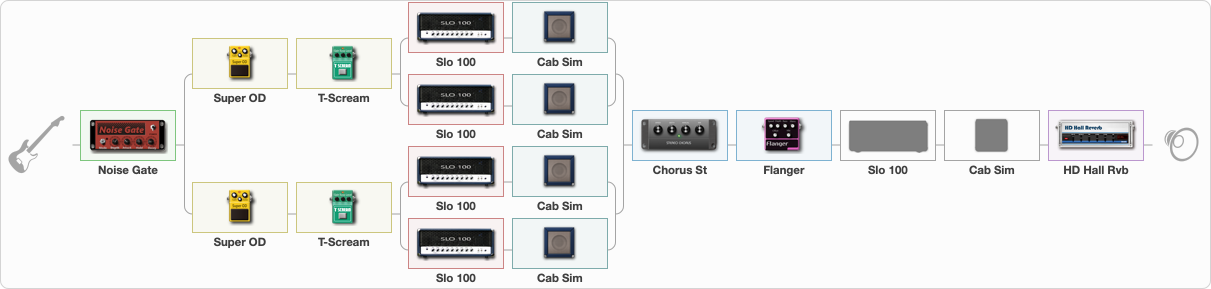# Dream State Clean

Discussion in 'ToneLib-GFX presets' started by davidtaylors, May 24, 2020.

1. ### davidtaylorsActive Member

Dream State Clean

Preset name: Dream State Clean

Clean Humbucker

Effects chain:Effect: "Noise Gate" (Dynamics / Filter), active - "yes"
{
"Mode" = Auto
"Depth" = 50
"Threshold" = 50
"Attack" = 0
"Hold" = 5
"Decay" = 250
}

Effect: "Splitter" (Dynamics / Filter), active - "no"
{
"A-Bypass" = Off
"A-Pan" = 0
"A-Level" = 55
"B-Bypass" = Off
"B-Pan" = 0
"B-Level" = 55

'A' branch:
{

Effect: "Super OD" (Overdrive / Distortion), active - "yes"
{
"Drive" = 8
"Tone" = 80
"Level" = 100
}

Effect: "T-Scream" (Overdrive / Distortion), active - "yes"
{
"Drive" = 22
"Tone" = 80
"Level" = 79
}

Effect: "Splitter" (Dynamics / Filter), active - "yes"
{
"A-Bypass" = Off
"A-Pan" = 0
"A-Level" = 55
"B-Bypass" = Off
"B-Pan" = 0
"B-Level" = 55

'A' branch:
{

Effect: "Slo 100" (Amp simulators), active - "yes"
{
"Gain" = 91
"Bass" = 22
"Middle" = 20
"Treble" = 72
"Presence" = 52
"Master" = 45
"Level (dB)" = -8
}

Effect: "Cab Sim" (Cabinets), active - "yes"
{
"Model" = 4x12" 1960 T75
"Level (dB)" = 2
}
}
'B' branch:
{

Effect: "Slo 100" (Amp simulators), active - "yes"
{
"Gain" = 81
"Bass" = 32
"Middle" = 40
"Treble" = 72
"Presence" = 62
"Master" = 45
"Level (dB)" = -4
}

Effect: "Cab Sim" (Cabinets), active - "yes"
{
"Model" = 4x12" Cali v30
"Level (dB)" = 4
}
}
}
}
'B' branch:
{

Effect: "Super OD" (Overdrive / Distortion), active - "yes"
{
"Drive" = 8
"Tone" = 80
"Level" = 100
}

Effect: "T-Scream" (Overdrive / Distortion), active - "yes"
{
"Drive" = 22
"Tone" = 80
"Level" = 79
}

Effect: "Splitter" (Dynamics / Filter), active - "yes"
{
"A-Bypass" = Off
"A-Pan" = 0
"A-Level" = 55
"B-Bypass" = Off
"B-Pan" = 0
"B-Level" = 55

'A' branch:
{

Effect: "Slo 100" (Amp simulators), active - "yes"
{
"Gain" = 91
"Bass" = 22
"Middle" = 20
"Treble" = 72
"Presence" = 52
"Master" = 45
"Level (dB)" = -4
}

Effect: "Cab Sim" (Cabinets), active - "yes"
{
"Model" = 4x12" 1960 T75
"Level (dB)" = 4
}
}
'B' branch:
{

Effect: "Slo 100" (Amp simulators), active - "yes"
{
"Gain" = 81
"Bass" = 32
"Middle" = 40
"Treble" = 82
"Presence" = 64
"Master" = 54
"Level (dB)" = -6
}

Effect: "Cab Sim" (Cabinets), active - "yes"
{
"Model" = 4x12" Cali v30
"Level (dB)" = 2
}
}
}
}
}

Effect: "Chorus St" (Modulation / Sfx), active - "yes"
{
"Speed" = 1.0
"Depth" = 97
"Center" = 10.0
"Mix" = 84
}

Effect: "Flanger" (Modulation / Sfx), active - "yes"
{
"Speed" = 0.8
"Depth" = 96
"Reso" = 9
"Center" = 5.6
"Offset" = 5.0
"Mix" = 100
"Reset" = On
}

Effect: "Slo 100" (Amp simulators), active - "no"
{
"Gain" = 91
"Bass" = 59
"Middle" = 34
"Treble" = 72
"Presence" = 86
"Master" = 50
"Level (dB)" = -1
}

Effect: "Cab Sim" (Cabinets), active - "no"
{
"Model" = 4x12" Cali v30
"Level (dB)" = 0
}

Effect: "HD Hall Rvb" (Reverb), active - "yes"
{
"Time" = 7.7
"PreLPF" = 44
"PreDelay" = 96
"HiDamp" = 27
"LoGain" = -3.3
"Mix" = 48
}

Note: You will need to download and install the ToneLib-GFX software to use the preset.

#### Attached Files:

• ###### Dream_State_Clean.tlgfx
File size:
1.8 KB
Views:
3,635
NarFi, SEMENOV, schllr and 9 others like this.